# AQA A Level Maths: Pure复习笔记4.1.1 Binomial Expansion

### Binomial Expansion

#### What is the binomial expansion?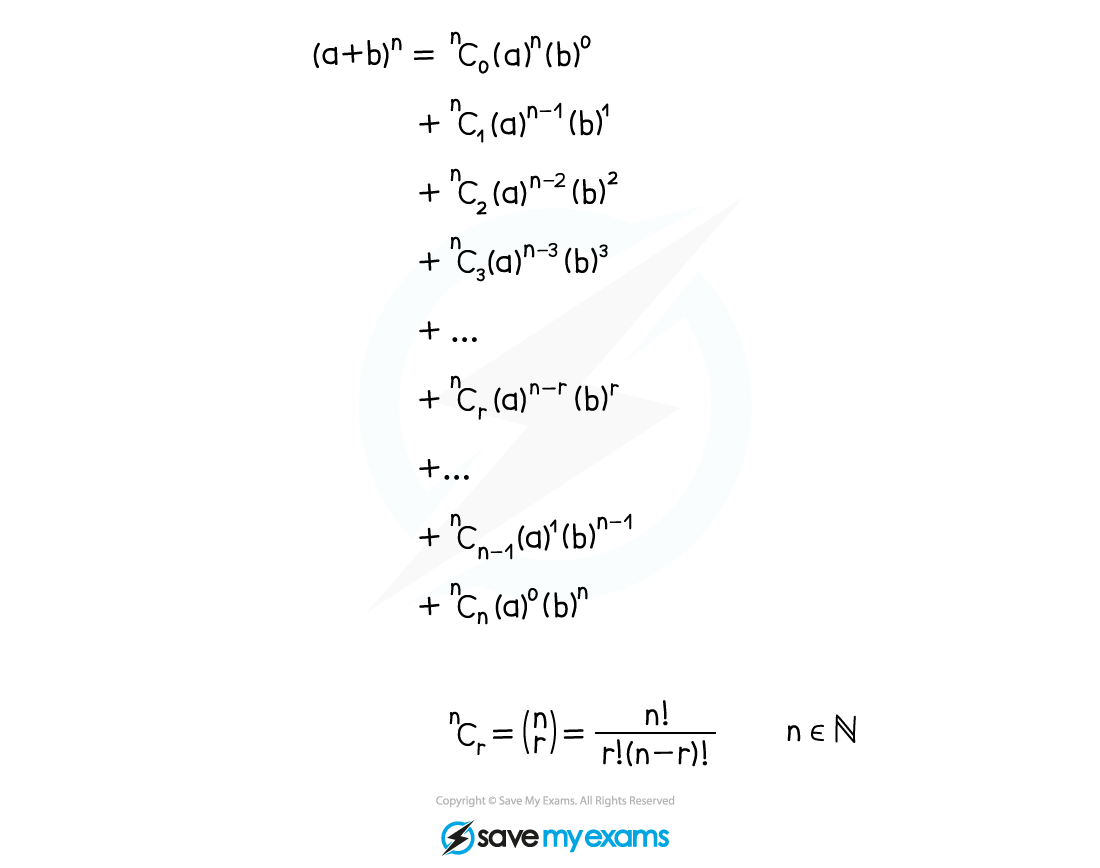• Look at the pattern
• Start at nC0, then nC1, nC2, etc
• Powers of a start at n and decrease by 1
• Powers of b start at 0 and increase by 1

• There are shortcuts but these hide the pattern
• nC0 = nCn = 1
• nC1 = nCn-1 = n
• nCr = nCn-r
• (b)0 = (a)0 = 1

• Use the shortcuts once familiar with the pattern
• ! means factorial• This is given in the formula booklet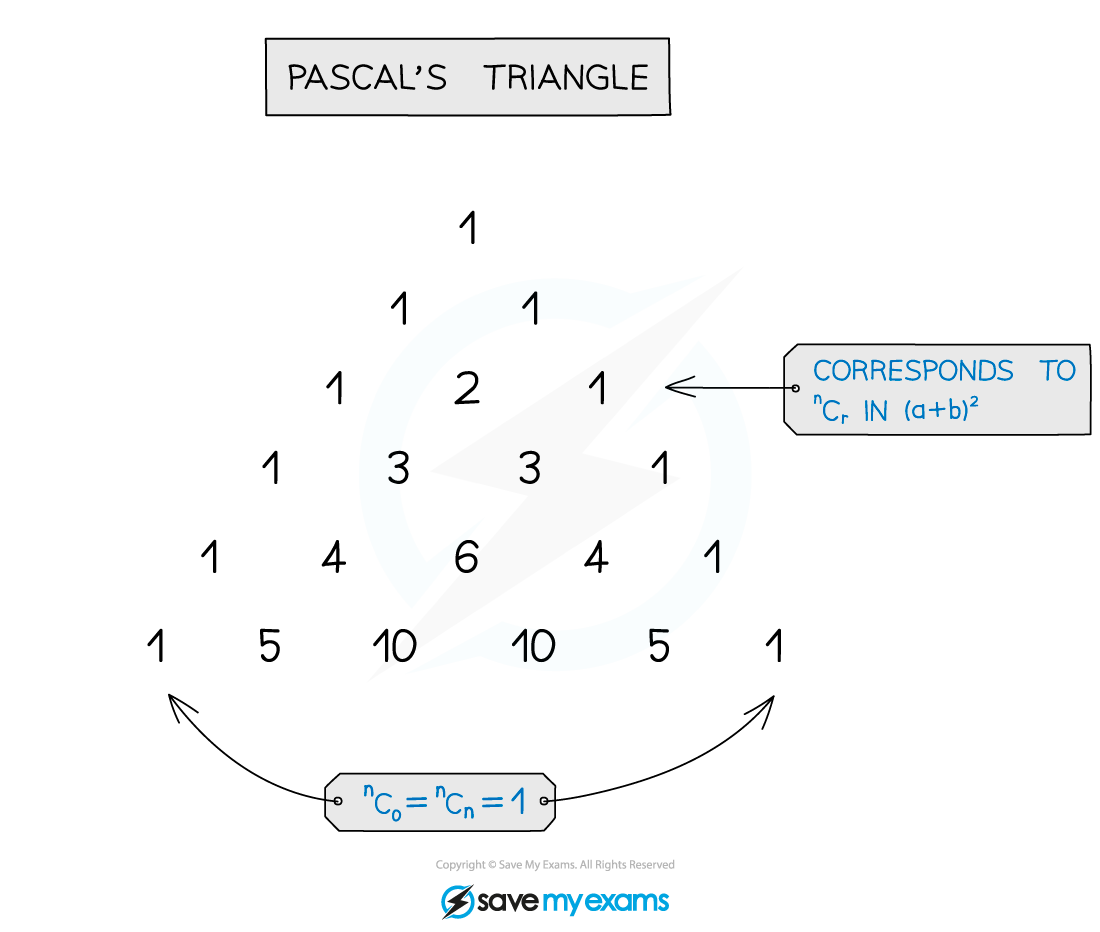• Pascal’s triangle is an alternative to nCr
• It is useful for lower values of n
• For larger n it is slow and prone to arithmetic errors

#### How do I expand brackets with binomial expansion?• Use a line for each term to make things easier to read and follow
• Use brackets, particularly helpful when negatives involved
• Use a calculator for nCr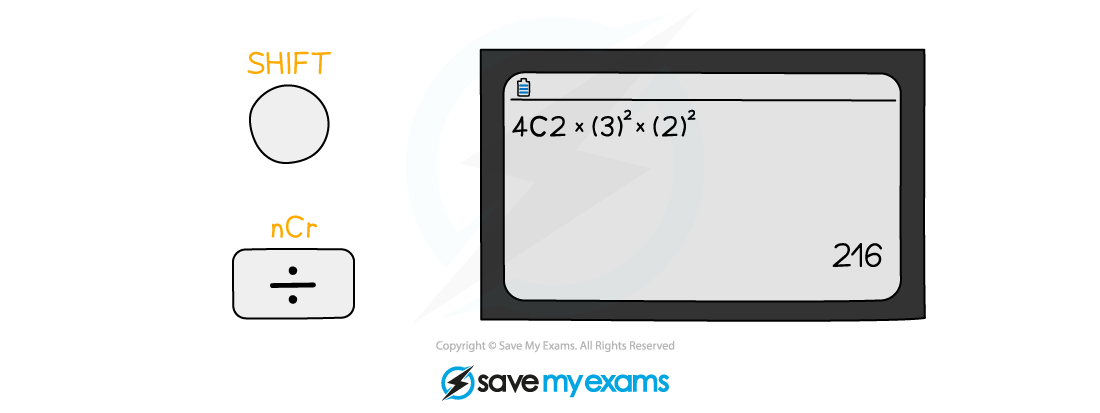#### What are binomial expansions used for?

• Expanding brackets, it is usual to be asked for either …
• … the first few terms only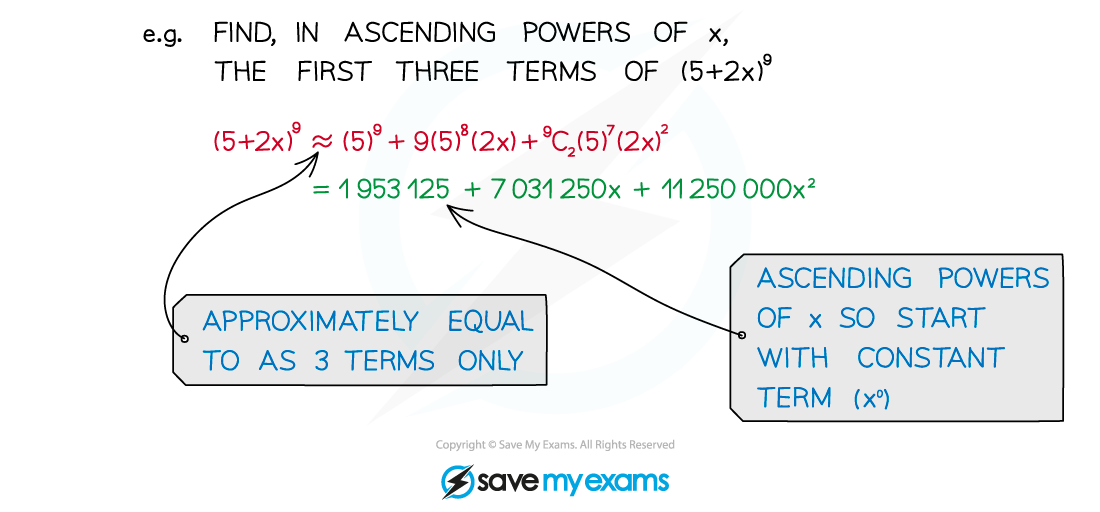• or (the coefficient of) a particular term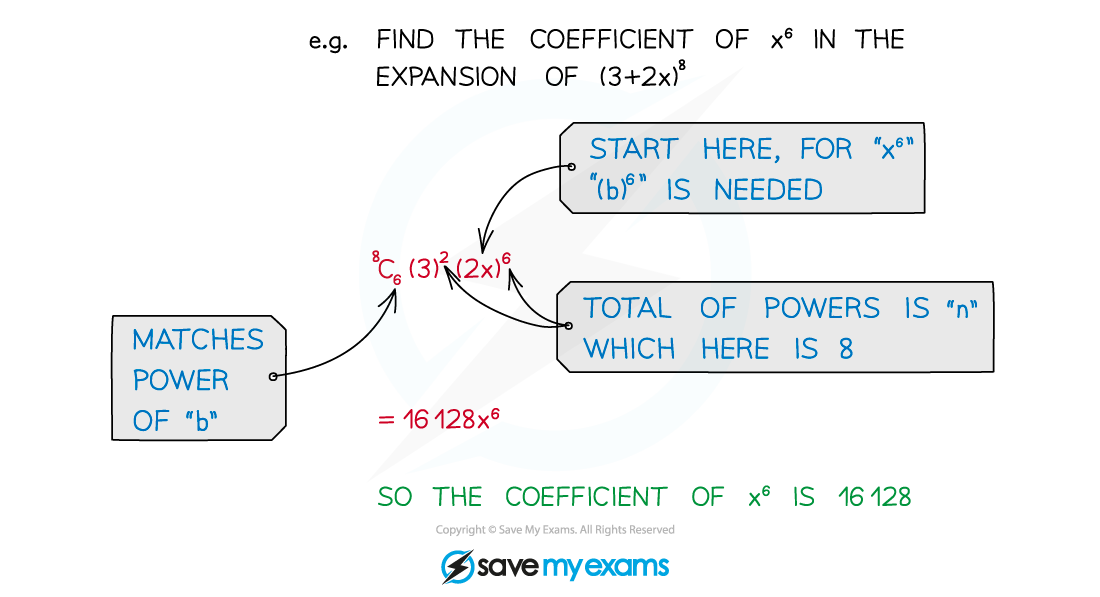• Solving problems in unknowns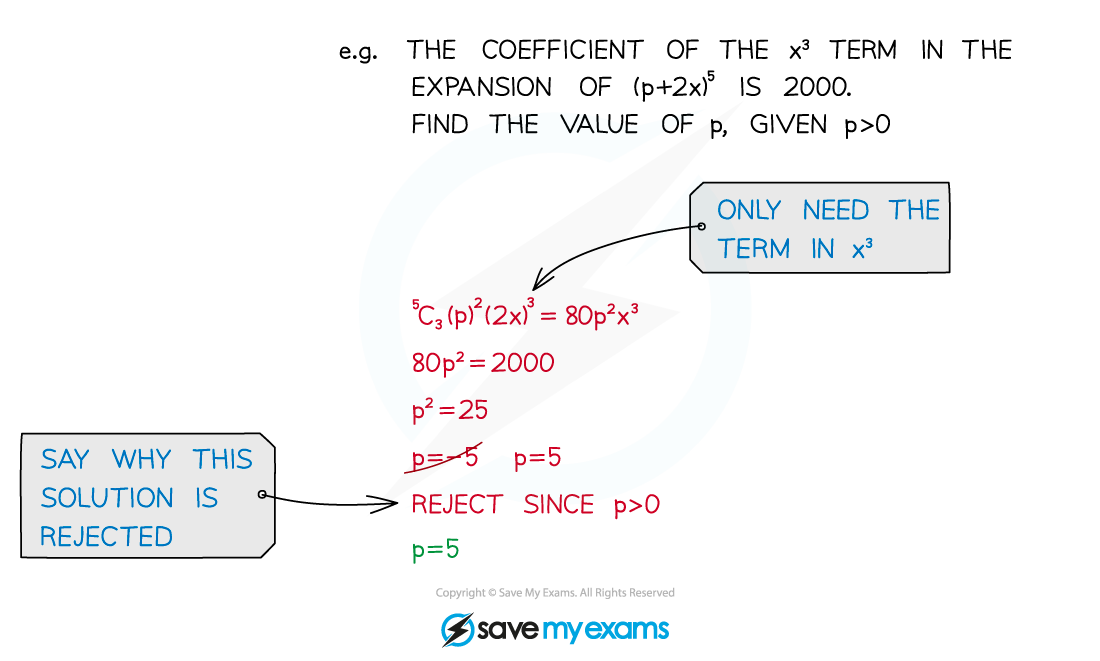#### Worked Example